Bloom Filter是一种空间效率很高的随机数据结构，它利用位数组很简洁地表示一个集合，并能判断一个元素是否属于这个集合。Bloom Filter的这种高效是有一定代价的：在判断一个元素是否属于某个集合时，有可能会把不属于这个集合的元素误认为属于这个集合（false positive）。通常应用在一些需要快速判断某个元素是否属于集合，但是并不严格要求100%正确的场合。而在能容忍低错误率的应用场合下，Bloom Filter通过极少的错误换取了存储空间的极大节省。

### 集合表示和元素查询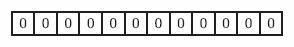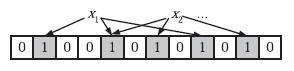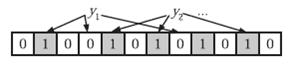### 错误率估计

$p'=(1-\frac{1}{m})^{kn} ≈ e^{-\frac{kn}{m}}$

$\lim_{x \to \infty}(1-\frac{1}{x})^{-x} = e$

$(1-ρ)^k ≈ (1-p')^k ≈ (1-p)^k$

$(1-ρ)$为位数组中1的比例，$(1-ρ)^k$就表示k次哈希都刚好选中1的区域，即false positive rate。上式中第二步近似在前面已经提到了，现在来看第一步近似。$p’$只是$ρ$的数学期望，在实际中$ρ$的值有可能偏离它的数学期望值。M. Mitzenmacher 已经证明 ，位数组中0的比例非常集中地分布在它的数学期望值的附近。因此，第一步的近似得以成立。分别将$p$和$p’$代入上式中，得：

$f' = (1-(1-\frac{1}{m})^{kn})^k = (1-p')^k$

$f = (1-e^{-\frac{kn}{m}})^k = (1-p)^k$

### 最优的哈希函数个数

$g = -\frac{m}{n}\ln(p)\ln(1-p)$

$g' = \frac{1}{n\ln(1-\frac{1}{m})}\ln(p')\ln(1-p')$

### 位数组的大小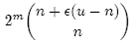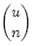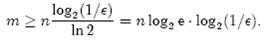### 参考文献